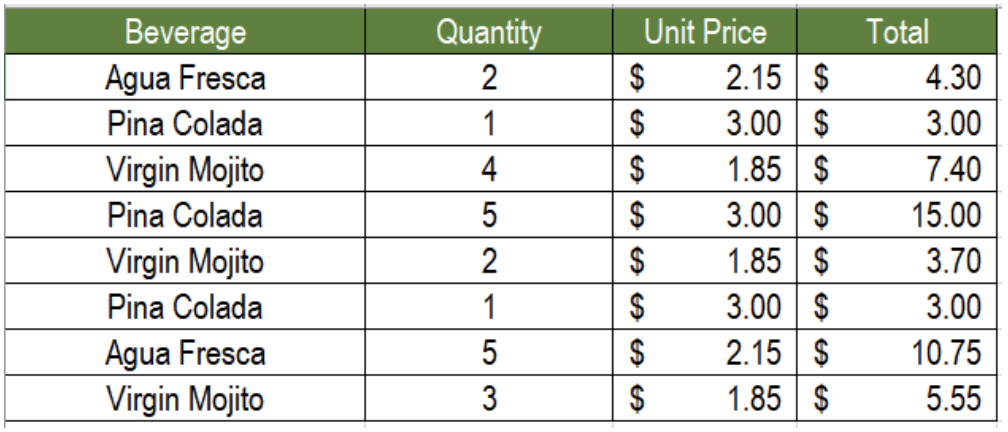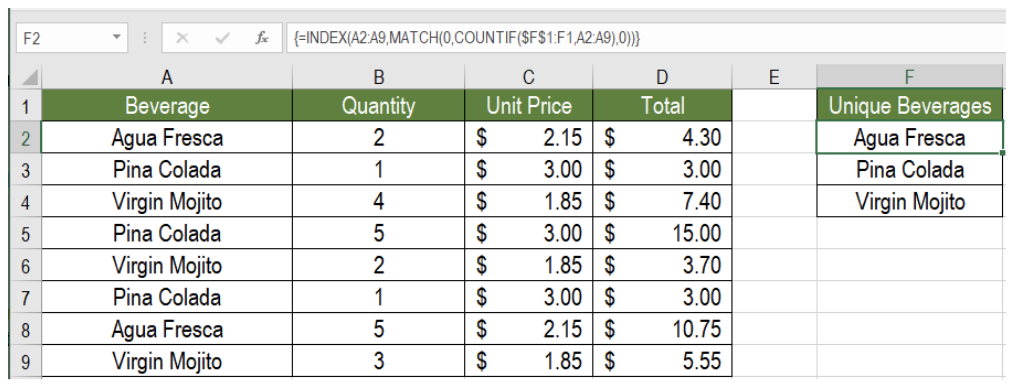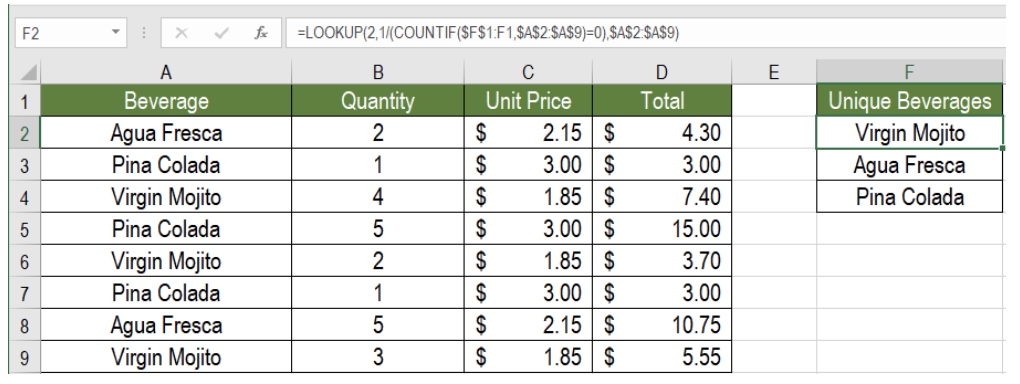Get instant live expert help with Excel or Google Sheets“My Excelchat expert helped me in less than 20 minutes, saving me what would have been 5 hours of work!”

#### Post your problem and you’ll get Expert help in seconds.

Your message must be at least 40 characters
Our professional Expert are available now. Your privacy is guaranteed.

# How to Extract Unique Items from a ListFigure 1. Sample Data Set to Extract Unique Names from list

We might come across a lot of duplicates while working with a large data set. Excel offers a few ways to extract the unique items from these. This tutorial shows how to extract unique items from a list.

## The Generic formula to Extract Unique Items from a List using an Array Formula

```=INDEX(list,MATCH(0,COUNTIF(exp_range,list),0)) ```
The formula consists of the functions INDEX, MATCH and COUNTIF. This is an array formula, hence, we need to press CTRL + SHIFT + ENTER to apply it. Here, the INDEX function is consisted of all the cells which has duplicates. We use the match Function to look for data with an absolute match. Then, COUNTIF returns 1 when there is a duplicate and 0 otherwise.

As we want to extract unique items from a list, we need to search and look for the value 0. This will work when we use the MATCH function. It provides the comparative positioning of the initial equivalent value of the array. Depending on this result, the INDEX formula will extract unique items from a list.

### Example

The following example contains a beverage sale data for a day. Column A contains the beverage names, column B has the quantity, column C has the price. To find the unique beverage names in column F, we need to:

• Go to cell F2. Click on it with your mouse to select it.
• Assign the formula `=INDEX(A2:A9,MATCH(0,COUNTIF(\$F\$1:F1,A2:A9),0))` to cell F2.
• Press CTRL + SHIFT + ENTER.
• Drag the formula using the fill handle from cells F2 to F4.Figure 2. How to Extract Unique Names from list Using an Array Formula

This will extract the unique beverage names in cells F2 to F4.

## The Generic Formula to Extract Unique Items from a List using a a Non-Array Formula

`=LOOKUP(2,1/(COUNTIF(exp_range,list)=0),list)`

Here, the COUNTIF function returns the counts of each value from the list, which is then compared to zeros. This in turns creates an array consisting of the values TRUE and FALSE. This array is then used as a divisor while 1 is kept as the dividend. The resulting array is consisted of the values 1 and  #DIV/0 error.

This is nested inside a LOOKUP function as the lookup_vector. 2 is the largest lookup value. So LOOKUP matches the last value that does not have an error and returns the corresponding value.

### Example

To extract unique values from the previous example using the LOOKUP function:

• We need to select cell F2.
• Assign the formula `=LOOKUP(2,1/(COUNTIF(\$F\$1:F1,\$A\$2:\$A\$9)=0),\$A\$2:\$A\$9) `to cell F2.
• Press ENTER.
• Drag the formula from cells F2 to F4.Figure 3. How to Extract Unique Names from list Using a Non-Array Formula

This formula will also retrieve the unique names of beverages in cells F2 to F4.

## Notes

Though there are some criteria which need to be fulfilled to use COUNTIF. To get the relative location of the result, the function MATCH is very effective. This function looks for the data which are absent in expanding the range.

Most of the time, the problems you will need to solve will be more complex than a simple application of a formula or function. If you want to save hours of research and frustration, try our live Excelchat service! Our Excel Experts are available 24/7 to answer any Excel question you may have. We guarantee a connection within 30 seconds and a customized solution within 20 minutes.

### Did this post not answer your question? Get a solution from connecting with the expert.Another blog reader asked this question today on Excelchat:
Solution examplesI need a formula to compare the data in two columns and then export the mismatched data in the 3rd column
Solved by S. Q. in 20 minsWe are trying to create a pivot table and combine two separate workbooks, that do not have the same data in the same cells. Is this even possible? For example the data is sales on items by month, one workbook has the sales broke down by month, the other work book has sales broke down by week. We can convert the data, before hand, but we are trying to forecast sales of times.
Solved by T. L. in 60 minsUse the Vlookup Function to complete the "employee" column of table 2. Use "job Id" from table 2 as your lookup_value(s) and table 1 as your reference.
Solved by C. H. in 16 minsI need to sum the number of hours per month one of three halls are being rented
Solved by A. F. in 60 minsVLOOKUP help. Trying to input data from database into new table
Solved by S. Q. in 40 mins## Subscribe to Excelchat.coAnother blog reader asked this question today on Excelchat: